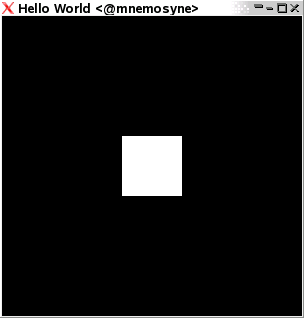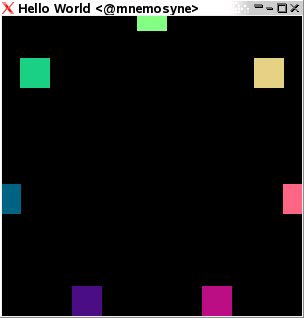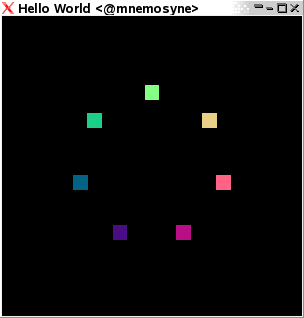# OpenGL programming in Haskell, a tutorial (Part 2)

Published: Tue 19 September 2006

As we left off the last installment, we were just about capable to open up a window, and draw some basic things in it by giving coordinate lists to the command renderPrimitive. The programs we built suffered under a couple of very infringing and ugly restraints when we wrote them - for one, they weren't really very modularized. The code would have been much clearer had we farmed out important subtasks on other modules. For another, we never even considered the fact that some manipulations would not necessarily be good to do on the entire picture.

## Some modules

To deal with the first problem, let's break apart our program a little bit, forming several more or less independent code files linked together to form a whole.

First off, HelloWorld.hs - containing a very generic program skeleton. We will use our module Bindings to setup everything else we might need, and tie them to the callbacks.

```import Graphics.Rendering.OpenGL
import Graphics.UI.GLUT
import Bindings

main = do
(progname,_) <- getArgsAndInitialize
createWindow "Hello World"
displayCallback \$= display
reshapeCallback \$= Just reshape
keyboardMouseCallback \$= Just keyboardMouse
mainLoop
```

Then Bindings.hs - our switchboard

```module Bindings (display,reshape,keyboardMouse) where
import Graphics.Rendering.OpenGL
import Graphics.UI.GLUT

import Display

reshape s@(Size w h) = do
viewport \$= (Position 0 0, s)

keyboardMouse key state modifiers position = return ()
```

We're going to be hacking around a LOT with the display function, so let's isolate that one to a module of it's own: Display.hs

```module Display (display) where
import Graphics.Rendering.OpenGL
import Graphics.UI.GLUT
import Cube

display = do
clear [ColorBuffer]
cube (0.2::GLfloat)
flush
```

And a first utility module, containing the gritty details of drawing the cube [tex][-w,w]^3[/tex], called Cube.hs

```module Cube where
import Graphics.Rendering.OpenGL
import Graphics.UI.GLUT

cube w = do
vertex \$ Vertex3 w w w
vertex \$ Vertex3 w w (-w)
vertex \$ Vertex3 w (-w) (-w)
vertex \$ Vertex3 w (-w) w

vertex \$ Vertex3 w w w
vertex \$ Vertex3 w w (-w)
vertex \$ Vertex3 (-w) w (-w)
vertex \$ Vertex3 (-w) w w

vertex \$ Vertex3 w w w
vertex \$ Vertex3 w (-w) w
vertex \$ Vertex3 (-w) (-w) w
vertex \$ Vertex3 (-w) w w

vertex \$ Vertex3 (-w) w w
vertex \$ Vertex3 (-w) w (-w)
vertex \$ Vertex3 (-w) (-w) (-w)
vertex \$ Vertex3 (-w) (-w) w

vertex \$ Vertex3 w (-w) w
vertex \$ Vertex3 w (-w) (-w)
vertex \$ Vertex3 (-w) (-w) (-w)
vertex \$ Vertex3 (-w) (-w) w

vertex \$ Vertex3 w w (-w)
vertex \$ Vertex3 w (-w) (-w)
vertex \$ Vertex3 (-w) (-w) (-w)
vertex \$ Vertex3 (-w) w (-w)
```

Now, compiling this entire section with the command

```ghc --make -package GLUT HelloWorld.hs -o HelloWorld
```

compiles and links each module needed, and produces, in the end, an executable to be used. There we go! Much more modularized, much smaller and simpler bits and pieces. And - an added boon - we won't normally need to recompile as much for each change we do.

This skeletal program will look like.

## Local transformations

One of the core reasons I started to write this tutorial series was that I wanted to figure out why Panitz' tutorial didn't work for me. The core explanation is simple - the names of some of the functions used has changed since he wrote them. Thus, the matrixExcursion in his tutorial is nowadays named preservingMatrix. This may well change further - though I hope it won't - in which case this tutorial will be painfully out of date as well.

The idea of preservingMatrix, however, is to take a small piece of drawing actions, and perform them independent of the transformations going on outside that small piece. For demonstration, let's draw a bunch of cubes, shall we?

We'll change the rather boring display subroutine in Display.hs into one using preservingMatrix to modify each cube drawn individually, giving a new Display.hs:

```module Display (display) where

import Graphics.Rendering.OpenGL
import Graphics.UI.GLUT

import Cube

points :: [(GLfloat,GLfloat,GLfloat)]
points = map (\k -> (sin(2*pi*k/12),cos(2*pi*k/12),0.0))  [1..12]

display = do
clear [ColorBuffer]
do mapM_ (\(x,y,z) -> preservingMatrix \$ do
color \$ Color3 x y z
translate \$ Vector3 x y z
cube (0.1::GLfloat)
) points
flush
```

Say... Those points on the unit circle might be something we'll want more of. Let's abstract some again! We'll break them out to a Points.hs. We'll have to juggle a bit with the typesystem to get things to work out, and in the end we get

```module Points where
import Graphics.Rendering.OpenGL

points :: Int -> [(GLfloat,GLfloat,GLfloat)]
points n' = let n = fromIntegral n' in map (\k -> let t = 2*pi*k/n in (sin(t),cos(t),0.0))  [1..n]

and then we get the Display.hs

module Display (display) where

import Graphics.Rendering.OpenGL
import Graphics.UI.GLUT

import Cube
import Points

display = do
clear [ColorBuffer]
mapM_ (\(x,y,z) -> preservingMatrix \$ do
color \$ Color3 ((x+1.0)/2.0) ((y+1.0)/2.0) ((z+1.0)/2.0)
translate \$ Vector3 x y z
cube (0.1::GLfloat)
) \$ points 7
flush
```
where we note that we need to renormalize our colours to get them within the interval [0,1] from the interval [-1,1] in order to get valid colour values. The program looks likeThe point of this yoga doesn't come apparent until you start adding some global transformations as well. So let's! We add the line

```scale 0.7 0.7 (0.7::GLfloat)
```
just after the clear [ColorBuffer], in order to scale the entire picture. As a result, we getWe can do this with all sorts of transformations - we can rotate the picture, skew it, move the entire picture around. Using preservingMatrix, we make sure that the transformations "outside" apply in the way we'd expect them to.

## Back to the callbacks

### Animation

A lot of the OpenGL programming is centered around the program being prepared to launch some sequenc when some event occurs. Let's use this to build a rotating version of our bunch of points up there. In order to do things over time, we're going to be using the global callbacks idleCallback and timerCallback. So, we'll modify the structure of our files a bit - starting from the top.

We'll need a new callback. And we'll also need a state variable of our own, which in turn needs to be fed to all functions that may need to use it. Incorporating these changes, we get a new HelloWorld.hs:

```import Graphics.Rendering.OpenGL
import Graphics.UI.GLUT
import Bindings
import Data.IORef

main = do
(progname,_) <- getArgsAndInitialize
createWindow "Hello World"
reshapeCallback \$= Just reshape
keyboardMouseCallback \$= Just keyboardMouse
angle <- newIORef 0.0
displayCallback \$= (display angle)
idleCallback \$= Just (idle angle)
mainLoop
```
Note the addition of an angle, and an idle. We need to feed the value of angle both to idle and to display, in order for them to use it accordingly. Now, we need to define idle somewhere - and since we keep all the bits and pieces we modify a LOT in display, let's put it in there.
Exporting it all the way requires us to change the first line of Bindings.hs to
```module Bindings (idle,display,reshape,keyboardMouse) where
```

Display.hs:

```module Display (display,idle) where

import Graphics.Rendering.OpenGL
import Graphics.UI.GLUT
import Data.IORef

import Cube
import Points

display angle = do
clear [ColorBuffer]
a <- get angle
rotate a \$ Vector3 0 0 (1::GLfloat)
scale 0.7 0.7 (0.7::GLfloat)
mapM_ (\(x,y,z) -> preservingMatrix \$ do
color \$ Color3 ((x+1.0)/2.0) ((y+1.0)/2.0) ((z+1.0)/2.0)
translate \$ Vector3 x y z
cube (0.1::GLfloat)
) \$ points 7
flush

idle angle = do
a <- get angle
angle \$= a + 0.1
```

Now, running this program makes a couple of different things painfully obvious. One is that things flicker. Another is that our ring is shrinking violently. The shrinking is due to our forgetting to reset all our transformations before we apply the next, and the flicker is because we're redrawing an entire picture step by step. Much smoother animation'll be had if we use a double buffering technique. Now, this isn't at all hard. We need to modify a few places - tell HOpenGL that we want to do doublebuffering and also when we want to swap the ready drawn canvas for the one on the screen. So, we modify, again, HelloWorld.hs:

```import Graphics.Rendering.OpenGL
import Graphics.UI.GLUT
import Data.IORef
import Bindings

main = do
(progname,_) <- getArgsAndInitialize
initialDisplayMode \$= [DoubleBuffered]
createWindow "Hello World"
reshapeCallback \$= Just reshape
keyboardMouseCallback \$= Just keyboardMouse
angle <- newIORef 0.0
idleCallback \$= Just (idle angle)
displayCallback \$= (display angle)
mainLoop
```

and we also need to modify Display.hs to implement the bufferswapping. While we're at it, we add the command loadIdentity, which resets the modification matrix.

```module Display (display,idle) where

import Graphics.Rendering.OpenGL
import Graphics.UI.GLUT
import Data.IORef

import Cube
import Points

display angle = do
clear [ColorBuffer]
a <- get angle
rotate a \$ Vector3 0 0 (1::GLfloat)
scale 0.7 0.7 (0.7::GLfloat)
mapM_ (\(x,y,z) -> preservingMatrix \$ do
color \$ Color3 ((x+1.0)/2.0) ((y+1.0)/2.0) ((z+1.0)/2.0)
translate \$ Vector3 x y z
cube (0.1::GLfloat)
) \$ points 7
swapBuffers

idle angle = do
a <- get angle
angle \$= a+0.1
postRedisplay Nothing
```

There we are! That looks pretty, doesn't it? Now, we could start adding control to the user, couldn't we? Let's add some keyboard interfaces. We'll start by letting the rotation direction change when we press spacebar, and let the arrows displace the whole figure and + and - increase/decrease the rotation speed.

Again, we're adding states, so we need to modify HelloWorld.hs

```import Graphics.Rendering.OpenGL
import Graphics.UI.GLUT
import Data.IORef
import Bindings

main = do
(progname,_) <- getArgsAndInitialize
initialDisplayMode \$= [DoubleBuffered]
createWindow "Hello World"
reshapeCallback \$= Just reshape
angle <- newIORef (0.0::GLfloat)
delta <- newIORef (0.1::GLfloat)
position <- newIORef (0.0::GLfloat, 0.0)
keyboardMouseCallback \$= Just (keyboardMouse delta position)
idleCallback \$= Just (idle angle delta)
displayCallback \$= (display angle position)
mainLoop
```

Note that position is sent along to the keyboard as well as the display callbacks. And in Bindings.hs, we give the keyboard callback actual function

```module Bindings (idle,display,reshape,keyboardMouse) where
import Graphics.Rendering.OpenGL
import Graphics.UI.GLUT
import Data.IORef

import Display

reshape s@(Size w h) = do
viewport \$= (Position 0 0, s)

keyboardAct a p (Char ' ') Down = do
a' <- get a
a \$= -a'
keyboardAct a p (Char '+') Down = do
a' <- get a
a \$= 2*a'
keyboardAct a p (Char '-') Down = do
a' <- get a
a \$= a'/2
keyboardAct a p (SpecialKey KeyLeft) Down = do
(x,y) <- get p
p \$= (x-0.1,y)
keyboardAct a p (SpecialKey KeyRight) Down = do
(x,y) <- get p
p \$= (x+0.1,y)
keyboardAct a p(SpecialKey KeyUp) Down = do
(x,y) <- get p
p \$= (x,y+0.1)
keyboardAct a p (SpecialKey KeyDown) Down = do
(x,y) <- get p
p \$= (x,y-0.1)
keyboardAct _ _ _ _ = return ()

keyboardMouse angle pos key state modifiers position = do
keyboardAct angle pos key state
```

finally, in Display.hs we use the new information to accordingly redraw the scene, specifically the now changing amount to change the current angle with. Note that in order to avoid the placement of the circle to be pulled in with all the other modifications we're doing, we do the translation outside a preservingMatrix call.

```module Display (display,idle) where

import Graphics.Rendering.OpenGL
import Graphics.UI.GLUT
import Data.IORef

import Cube
import Points

display angle position = do
clear [ColorBuffer]
(x,y) <- get position
translate \$ Vector3 x y 0
preservingMatrix \$ do
a <- get angle
rotate a \$ Vector3 0 0 (1::GLfloat)
scale 0.7 0.7 (0.7::GLfloat)
mapM_ (\(x,y,z) -> preservingMatrix \$ do
color \$ Color3 ((x+1.0)/2.0) ((y+1.0)/2.0) ((z+1.0)/2.0)
translate \$ Vector3 x y z
cube (0.1::GLfloat)
) \$ points 7
swapBuffers

idle angle delta = do
a <- get angle
d <- get delta
angle \$= a+d
postRedisplay Nothing
```

## Summary

We now know how to modify only parts of a picture, and we also know how to use the idle and the keyboardMouse callback to support animations and keyboard input.

In order to somewhat limit the amount of typing I need to do, I'll give links that give details on some of the themes we've touched upon.

First of all, the callbacks are described in more detail and with call signatures at